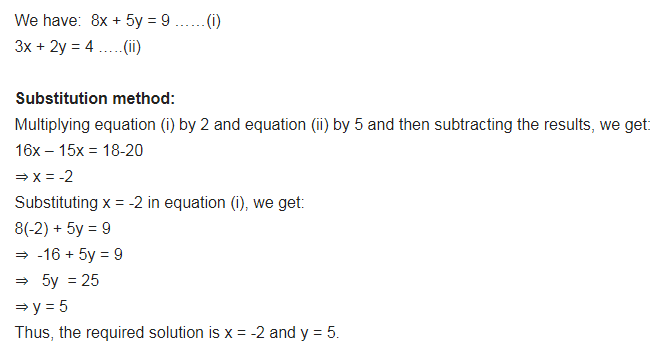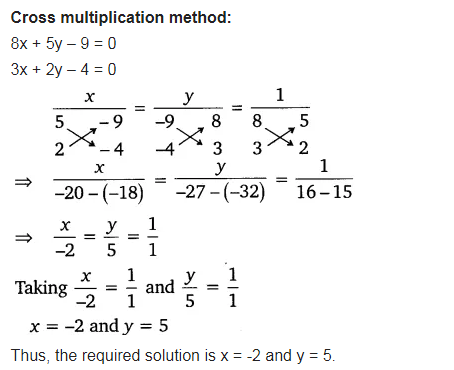# Solve the following pair of linear equations by the substitution and cross-multiplication methods:

Solve the following pair of linear equations by the substitution and cross-multiplication methods:
8x + 5y = 9, 3x + 2y = 4